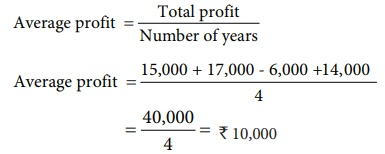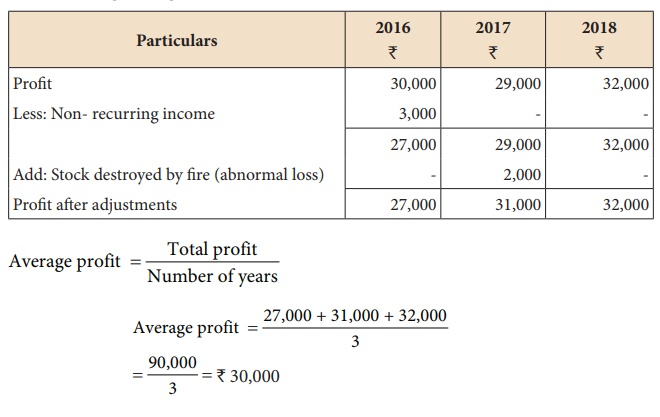Home | | Accountancy 12th Std | Methods of valuation of goodwill

# Methods of valuation of goodwill

Following are the methods generally followed to value goodwill:

Methods of valuation of goodwill

Following are the methods generally followed to value goodwill:

## 1. Average profit method

Under this method, goodwill is calculated as certain years of purchase of average profits of the past few years. The number of years of purchase is generally determined on the basis of the average period a new business will take in order to bring it to the current state of the existing business. It may also be determined on the basis of the number of years for which the past average profit will be earned in future.

While computing the average profit, in addition to rectification of errors, the following adjustments are to be made:

Adjusted profit = Actual profit + Past expenses not required in the future – Past revenues not likely to be earned in the future + Additional income expected in the future – Additional expenses expected to be paid in the future

The average profit may be either simple average profit or weighted average profit.

## (a) Simple average profit method

Under this method, goodwill is calculated by multiplying the average profits by a certain number of years of purchase. Simple average profit is calculated by adding the adjusted profits of certain number of years by dividing the total number of such years.

Goodwill = Average profit × Number of years of purchase

Average profit = Total profit / Number of yearsIllustration 1

The following are the profits of a firm in the last five years:

2014: 4,000; 2015: 3,000;  2016: 5,000;  2017: 4,500 and 2018: 3,500 Calculate the value of goodwill at 3 years purchase of average profits of five years.

Solution

Goodwill = Average profit × Number of years of purchaseGoodwill = Average profit × Number of years of purchase

= 4,000 × 3 = ₹ 12,000

Illustration 2

The profits and losses of a firm for the last four years were as follows:

2015: 15,000;  2016: 17,000;  2017: 6,000 (Loss); 2018: 14,000

You are required to calculate the amount of goodwill on the basis of 5 years purchase of average profits of the last 4 years.

Solution

Goodwill = Average profit × Number of years of purchase

Average profit = Total profit / Number of yearsGoodwill = Average profit × Number of years of purchase

= 10,000 × 5 = ₹ 50,000

Illustration 3

A partnership firm has decided to value its goodwill for the purpose of settling a retiring partner. The profits of that firm for the last four years were as follows:

2015: 40,000; 2016: 50,000; 2017: 48,000 and 2018: 46,000

The business was looked after by a partner. No remuneration was paid to him. The fair remuneration of the partner valued at comes to 6,000 per annum.

Find out the value of goodwill, if it is valued on the basis of three years purchase of the average profits of the last four years.

SolutionGoodwill = Average profit × Number of years of purchase

= 40,000 × 3 = ₹ 1,20,000

Illustration 4

From the following information relating to Arul enterprises, calculate the value of goodwill on the basis of 2 years purchase of the average profits of 3 years.

a)     Profits for the years ending 31st December 2016, 2017 and 2018 were 46,000, 44,000 and 50,000 respectively.

b)    A non-recurring income of 5,000 is included in the profits of the year 2016.

c)     The closing stock of the year 2017 was overvalued by 10,000.

SolutionTutorial note: Over valuation of closing stock in 2017 will result in over valuation of opening stock in 2018Goodwill = Average profit × Number of years of purchase = 45,000 × 2 = ₹ 90,000

Illustration 5

The following particulars are available in respect of a business carried on by a partnership firm:

a)     Profits earned: 2016: 30,000; 2017: 29,000 and 2018: 32,000.

b)    Profit of 2016 includes a non-recurring income of 3,000.

c)     Profit of 2017 is reduced by 2,000 due to stock destroyed by fire.

d)    The stock is not insured. But, it is decided to insure the stock in future. The insurance premium is estimated at 5,600 per annum.

You are required to calculate the value of goodwill on the basis of 2 years purchase of average profits of the last three years.

Solution

### (a) Calculation of adjusted profitGoodwill = Average profit × Number of years of purchase

= 24,400 × 2

= ₹ 48,800

### (b) Weighted average profit method

Under this method, goodwill is calculated by multiplying the weighted average profit by a certain number of years of purchase.

Goodwill = Weighted average profit × Number of years of purchase

In this method, weights are assigned to each year’s profit. Weighted profit is ascertained by multiplying the weights assigned with the respective year’s profit. The sum of the weighted profits is divided by the sum of weights assigned to determine the weighted average profit.

Weighted average profit = Total of weighted profits / Total of weightsThis method is used when the profits show an increasing or decreasing trend. More weight is generally given to the profits of the recent years.

Illustration 6

For the purpose of admitting a new partner, a firm has decided to value its goodwill at 3 years purchase of the average profit of the last 4 years using weighted average method. Profits of the past 4 years and the respective weights are as follows:Compute the value of goodwill.

Solution

Calculation of weighted average profitGoodwill = Weighted average profit × Number of years of purchase

= 24,800 × 3 = ₹ 74,400

## 2. Super profit methods

Under these methods, super profit is the base for calculation of the value of goodwill. Super profit is the excess of average profit over the normal profit of a business.

Super profit = Average profit – Normal profit

Average profit is calculated by dividing the total of adjusted actual profits of certain number of years by the total number of such years. Normal profit is the profit earned by the similar business firms under normal conditions.

Normal profit       = Capital employed × Normal rate of return

Capital employed = Fixed assets + Current assets – Current liabilities

Normal rate of return  = It is the rate at which profit is earned by similar business entities in the industry under normal circumstances.

## (a) Purchase of super profit method

Under this method, goodwill is calculated by multiplying the super profit by a certain number of years of purchase.

Goodwill = Super profit × Number of years of purchase

Illustration 7

From the following information, calculate the value of goodwill based on 3 years purchase of super profit

i.            Capital employed: 2,00,000

ii.            Normal rate of return: 15%

iii.            Average profit of the business: 42,000

Solution

Normal profit = Capital employed × Normal rate of return

= 2,00,000 × 15% = ₹ 30,000

Super profit = Average profit – Normal profit

= 42,000 – 30,000

= ₹ 12,000

Goodwill = Super profit × Number of years of purchase

= 12,000 × 3

= ₹ 36,000

Illustration 8

Calculate the value of goodwill at 5 years purchase of super profit from the following information:

(a) Capital employed: 1,20,000

(b) Normal rate of profit: 20%

(c) Net profit for 5 years:

2014: 30,000; 2015: 32,000; 2016: 35,000; 2017: 37,000 and 2018: 40,000

(d) Fair remuneration to the partners 2,800 per annum.

SolutionNormal profit = Capital employed × Normal rate of return

= 1,20,000 × 20%

= ₹ 24,000

Super profit         = Average profit – Normal profit

= 32,000 – 24,000

= ₹ 8,000

Goodwill     = Super profit × Number of years of purchase

= 8,000 × 5

= ₹ 40,000

## (b) Annuity method

Under this method, value of goodwill is calculated by multiplying the super profit with the present value of annuity.

Goodwill = Super profit × Present value annuity factor

Present value annuity factor is the present value of annuity of rupee one at a given time. It can be found out from annuity table or by using formula.

Illustration 9

From the following information, compute the value of goodwill as per annuity method:

(a) Capital employed: 50,000

(b) Normal rate of return: 10%

(c) Profits of the years 2016, 2017 and 2018 were 13,000, 15,000 and 17,000 respectively.

The present value of annuity of 1 for 3 years at 10% is 2. 4868.

Solution

Average profit = Total profit / Number of years

= 13,000 + 15,000 + 17,000 / 3

= 45,000/3

= ₹ 15,000

Normal profit = Capital employed × Normal rate of return = 50,000 × 10% = ₹ 5,000

Super profit = Average profit – Normal profit = 15,000 – 5,000

= ₹ 10,000

Goodwill = Super profit × Value of annuity = 10,000 × 2.4868 = ₹ 24,868

## c. Capitalisation of super profit method

Under this method, value of goodwill is calculated by capitalising the super profit at normal rate of return, that is, goodwill is the capitalised value of super profit.

Goodwill = [ Super profit / Normal rate of return ] × 100

Illustration 10

From the following information, compute the value of goodwill by capitalising super profit:

a)     Capital employed is 4,00,000

b)    Normal rate of return is 10%

c)     Profit for 2016: 62,000; 2017: 61,000 and 2018: 63,000

Solution

Average profit = Total profit/Number of years

= [62,000 + 61,000 + 63,000] /3

= ₹ 62,000

Normal profit = Capital employed × Normal rate of return

= 4,00,000 × 10%

= ₹ 40,000

Super profit = Average profit – Normal profit

= 62,000 – 40,000

= ₹ 22,000

Goodwill = Super profit/Normal rate of return x 100

= 22,000/10 x 100

= ₹ 2,20,000

## 3. Capitalisation method

Under this method, goodwill is the excess of capitalised value of average profit of the business over the actual capital employed in the business.

Goodwill = Total capitalised value of the business – Actual capital employed

The total capitalised value of the business is calculated by capitalising the average profits on the basis of the normal rate of return.

Capitalised value of the business = [ Average profit / Normal rate of return ]  x 100

Actual capital employed = Fixed assets (excluding goodwill) + Current assets – Current liabilities

Illustration 11

From the following information, find out the value of goodwill by capitalisation method:

(a) Average profit = ₹ 60,000

(b) Normal rate of return         = 10%

(c) Capital employed     = ₹ 4,50,000

Solution

Total capitalised value of the average profit = [ Average profit / Normal rate of return ] x 100

= 60,000 / 10 = x 100

Goodwill = Total capitalised value of the average profit – Capital employed

= 6,00,000 – 4,50,000

= ₹ 1,50,000

Tags : Goodwill in Partnership Accounts | Accountancy , 12th Accountancy : Chapter 4 : Goodwill in Partnership Accounts
Study Material, Lecturing Notes, Assignment, Reference, Wiki description explanation, brief detail
12th Accountancy : Chapter 4 : Goodwill in Partnership Accounts : Methods of valuation of goodwill | Goodwill in Partnership Accounts | Accountancy